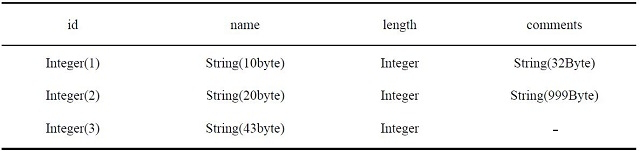Search
Document Center

# How does Table Store manage data storage?

Last Updated: Apr 11, 2018

Table Store charges fees per hour based on the total volume of instance data. Because a user’s data size can change dynamically, Table Store calculates a table’s data size at set time intervals, and then calculates the hourly average data size. The fee is calculated by multiplying the average data size by the unit price. The unit price may be updated by Alibaba Cloud. For the latest billing information, see Pricing.

An instance’s total data volume is the sum of the data from each table in the instance, and a table’s data volume is the sum of the data from all rows in the table. The following example explains how a table’s data volume is calculated.

In the following table, the ID is the primary key column, and all other columns are attribute columns:• For the row id=1, its data volume is: len (‘id’) + len (‘name’) + len (‘length’) + len (‘comments’) + 8 Byte + 10 Byte + 8 Byte + 32 Byte = 78 Byte.

• For the row id=2, its data volume is: len (‘id’) + len (‘name’) + len (‘length’) + len (‘comments’) + 8 Byte + 20 Byte + 8 Byte + 999 Byte = 1055 Byte.

• For the row id=3, its data size is: len (‘id’) + len (‘name’) + len (‘length’) + 8 Byte + 43 Byte + 8 Byte = 71 Byte.

The table’s data volume is 78 Byte + 1055 Byte + 71 Byte = 1204 Byte. If the table’s data volume does not change within the measured hour, the user is billed for 1,204 Bytes. Table Store does not limit the data volume of individual tables, nor does it charge for unused services, so it has no hidden costs.